# Distinguishing between the area and perimeter of a rectangle Online Quiz

#### Complete Python Prime Pack

9 Courses     2 eBooks

#### Artificial Intelligence & Machine Learning Prime Pack

6 Courses     1 eBooks

#### Java Prime Pack

9 Courses     2 eBooks

Following quiz provides Multiple Choice Questions (MCQs) related to Distinguishing between the area and perimeter of a rectangle. You will have to read all the given answers and click over the correct answer. If you are not sure about the answer then you can check the answer using Show Answer button. You can use Next Quiz button to check new set of questions in the quiz.Q 1 - Find the perimeter of the following rectangle.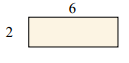### Explanation

Step 1:

Perimeter of a rectangle = 2(l + w); l = length = 6; w = width = 2

Step 2:

Perimeter of given rectangle = 2(6 + 2) = 16 units

Q 2 - Find the area of the following rectangle.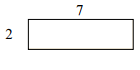### Explanation

Step 1:

Area of a rectangle = l × w; l = length = 7; w = width = 2

Step 2:

Area of given rectangle = 7 × 2 = 14 square units

Q 3 - Find the perimeter of the following rectangle.### Explanation

Step 1:

Perimeter of a rectangle = 2(l + w); l = length = 6; w = width = 5

Step 2:

Perimeter of given rectangle = 2(6 + 5) = 22 units

Q 4 - Find the area of the following rectangle.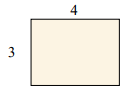### Explanation

Step 1:

Area of a rectangle = l × w; l = length = 4; w = width = 3

Step 2:

Area of given rectangle = 4 × 3 = 12 square units

Q 5 - Find the perimeter of the following rectangle.### Explanation

Step 1:

Perimeter of a rectangle = 2(l + w); l = length = 8; w = width = 7

Step 2:

Perimeter of given rectangle = 2(8 + 7) = 30 units

Q 6 - Find the area of the following rectangle.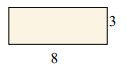### Explanation

Step 1:

Area of a rectangle = l × w; l = length = 8; w = width = 3

Step 2:

Area of given rectangle = 8 × 3 = 24 square units

Q 7 - Find the perimeter of the following rectangle.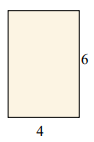### Explanation

Step 1:

Perimeter of a rectangle = 2(l + w); l = length = 6; w = width = 4

Step 2:

Perimeter of given rectangle = 2(6 + 4) = 20 units

Q 8 - Find the area of the following rectangle.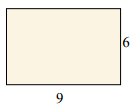### Explanation

Step 1:

Area of a rectangle = l × w; l = length = 9; w = width = 6

Step 2:

Area of given rectangle = 9 × 6 = 54 square units

Q 9 - Find the perimeter of the following rectangle.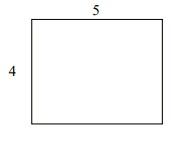### Explanation

Step 1:

Perimeter of a rectangle = 2(l + w); l = length = 5; w = width = 4

Step 2:

Perimeter of given rectangle = 2(5 + 4) = 18 units

Q 10 - Find the area of the following rectangle.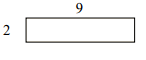### Explanation

Step 1:

Area of a rectangle = l × w; l = length = 9; w = width = 2

Step 2:

Area of given rectangle = 9 × 2 = 18 square units

distinguishing_between_area_and_perimeter_of_rectangle.htm